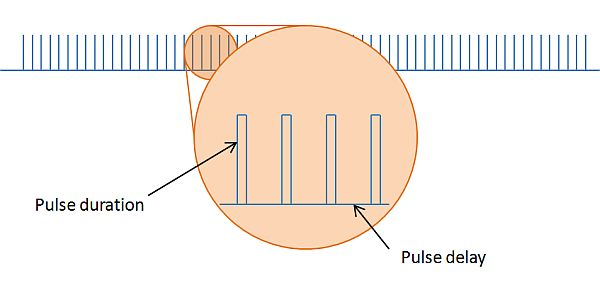# Conversion

The following conversion tables I use to understand foreign
dimensions and make it imaginable for me.

1 oz.(ounce) = 28,35 g.
1 lb.(pound) = 453,59 g.
1 in.(inch) = 25,40 mm
1 ft.(foot) = 0,30 m
1 yd.(yard) = 0,91 m
1 m.(mile) = 1,61 km
1 g. = 0,04 oz.(ounce)
1 kg = 2,22 lb.(pound)
1 mm = 0,04 in.(inch)
1 m = 3,3 ft.(foot)
1 m = 1,09 yd.(yard)
1 km = 0,62 m.(mile)